## Permutations and Combinations Questions and Answers Part-2

1. The exponent of 7 in $^{100}C_{50}$ is
a) 0
b) 2
c) 3
d) 4

Explanation: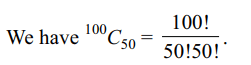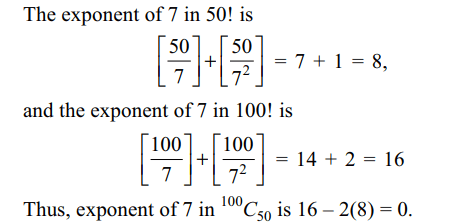2. In a certain test there are n questions. In this test $2^{k}$ students gave wrong answers to at least (n – k) questions, where k = 0, 1, 2, …, n. If the total number of wrong answers is 4095, then value of n is
a) 11
b) 12
c) 13
d) 15

Explanation: The number of students answering at least r3.If n > 1 and n divides (n – 1)! + 1, then
a) n must be prime
b) n must be divisible by exactly two primes
c) n must be a composite number
d) n must be divisible by $p^{2}$ where p is primes

Explanation: If n is not prime, then there exists r ∈ N such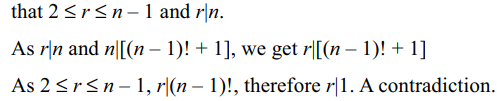4. A $11\times11$  grid is divided into five rectangles along the grid. The number of ways to divide the grid into five rectangles so that one of the rectangles generated by the division doesn’t have any edge on the periphery is
a) 32400
b) 16200
c) 14000
d) 18000

Explanation: There are 12 horizontal and 12 vertical lines on a 11 * 11 grid.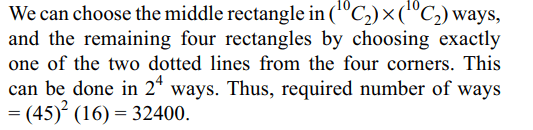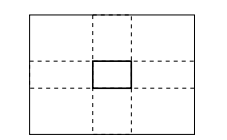5. For $n\geq 2$  , let $\alpha= \sum_{r=1}^{n}r!\sum_{s=1}^{n}s!\sqrt{s}\log_{10}\left(s\right)$       and $\beta=\sum_{r=1}^{n}r!\sqrt{r}\sum_{s=1}^{n}s!\log_{10}\left(s\right)$      , then
a) $\alpha=\beta$
b) $\alpha\beta=1$
c) $\alpha>\beta$
d) $\alpha<\beta$

Explanation:6. Sum of the series$\sum_{r=1}^{n}\left(r^{2}+1\right)\left(r!\right)$
is
a) $\left(n+1\right)!$
b) $\left(n+2\right)!-1$
c) $n\left(n+1\right)!$
d) $n\left(n+2\right)!$

Explanation:7. In a group of 8 girls, two girls are sisters. The number of ways in which the girls can sit so that two sisters are not sitting together is
a) 48200
b) 14100
c) 28300
d) 30240

Explanation: The required number of ways = the number of ways in which 8 girls can sit – the number of ways in which two sisters are together
= 8! – (2) (7!) = 30240.

8.The number of words that can be formed by using the letters of the word MATHEMATICS that start as well as end with T is
a) 80720
b) 90720
c) 20860
d) 37528

Explanation: The word MATHEMATICS contains 11 letters viz. M, M, A, A, T, T, H, E, I, C, S. The number of words that begin with T and end with T is9. If $a_{n}=\sum_{r=0}^{n}\frac{1}{^{n}C_{r}}$    , then $\sum_{r=0}^{n}\frac{r}{^{n}C_{r}}$    equals
a) $\frac{n}{2}a_{n}$
b) $\frac{n}{4}a_{n}$
c) $na_{n}$
d) $\left(n-1\right)a_{n}$

Explanation: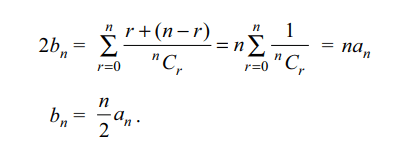10. m men and w women are to be seated in a row so that no two women sit together. If m > w, then the number of ways in which they can be seated is
a) $\frac{m!\left(m+1\right)!}{\left(m-w+1\right)!}$
b) $^{m}C_{m-w}\left(m-w\right)!$
c) $^{m+w}C_{m}\left(m-w\right)!$
d) none of these